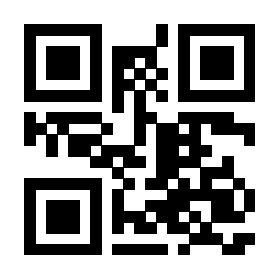# 关于使用 foreach 语法在 tikz 中绘制 node 的一个问题

\begin{tikzpicture}

\foreach[count=\m] \x in{0,2}
\foreach[count=\n] \y in{0,-1}
{\coordinate(a\n\m)at(\x,\y);}
\foreach \x/\y in{
a11/A,a12/B,
a21/C,a22/D}
{\node at(\x){\y};}

\end{tikzpicture}

0

438
1 个回答

### 撰写答案